MathScore EduFighter is one of the best math games on the Internet today. You can start playing for free!

Compare Integers - Sample Math Practice Problems

The math problems below can be generated by MathScore.com, a math practice program for schools and individual families. References to complexity and mode refer to the overall difficulty of the problems as they appear in the main program. In the main program, all problems are automatically graded and the difficulty adapts dynamically based on performance. Answers to these sample questions appear at the bottom of the page. This page does not grade your responses.

See some of our other supported math practice problems.

Complexity=5, Mode=v

Use >, <, or = to relate the two numbers.

 1.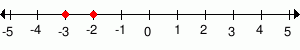-3 --- > < = -2 2.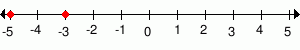-3 --- > < = -5

Complexity=10, Mode=v

Use >, <, or = to relate the two numbers.

 1.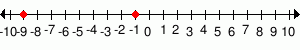-1 --- > < = -9 2.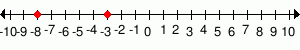-3 --- > < = -8

Complexity=5

Use >, <, or = to relate the two numbers.

 1.   -3 --- > < = -1 2.   -5 --- > < = -1

Complexity=10

Use >, <, or = to relate the two numbers.

 1.   -2 --- > < = 1 2.   -8 --- > < = -10

Complexity=5, Mode=v

Use >, <, or = to relate the two numbers.

1-3 -2
Solution
As you move left of the number line, the numbers become smaller in value.
As you move right of the number line, the numbers become greater in value.

-3 is to the left of -2.
This means that -3 < -2.
2-3 -5
Solution
As you move left of the number line, the numbers become smaller in value.
As you move right of the number line, the numbers become greater in value.

-3 is to the right of -5.
This means that -3 > -5.

Complexity=10, Mode=v

Use >, <, or = to relate the two numbers.

1-1 -9
Solution
As you move left of the number line, the numbers become smaller in value.
As you move right of the number line, the numbers become greater in value.

-1 is to the right of -9.
This means that -1 > -9.
2-3 -8
Solution
As you move left of the number line, the numbers become smaller in value.
As you move right of the number line, the numbers become greater in value.

-3 is to the right of -8.
This means that -3 > -8.

Complexity=5

Use >, <, or = to relate the two numbers.

1-3 -1
Solution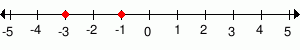As you move left of the number line, the numbers become smaller in value.
As you move right of the number line, the numbers become greater in value.

-3 is to the left of -1.
This means that -3 < -1.
2-5 -1
Solution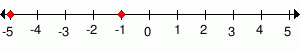As you move left of the number line, the numbers become smaller in value.
As you move right of the number line, the numbers become greater in value.

-5 is to the left of -1.
This means that -5 < -1.

Complexity=10

Use >, <, or = to relate the two numbers.

1-2 1
Solution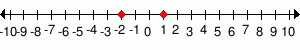As you move left of the number line, the numbers become smaller in value.
As you move right of the number line, the numbers become greater in value.

-2 is to the left of 1.
This means that -2 < 1.
2-8 -10
Solution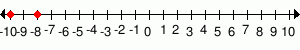As you move left of the number line, the numbers become smaller in value.
As you move right of the number line, the numbers become greater in value.

-8 is to the right of -10.
This means that -8 > -10.